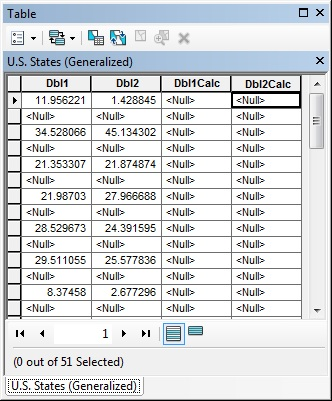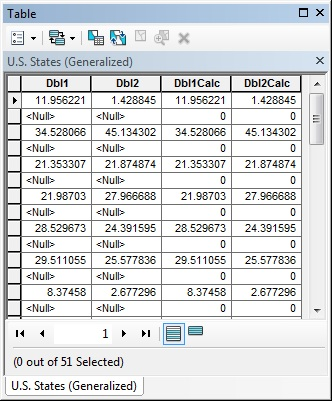HOW TO

# Calculate fields where some inputs are null values

Last Published: April 25, 2020

## Summary

These instructions provide examples of VBScript and Python syntax that show a way to calculate fields where some input values are null.

```Note:
Be aware that the Python form of the script produces the expected results on 10.1, but on 10.0 does not return a value for null inputs. VBScript produces the correct results on both versions.```

## Procedure

The calculation in each case applies the source value (in this case, the field Dbl1 or Dbl2) to the target field unless the source is null, in which case the target field is assigned the value zero.

VBScript:

1. Copy and paste the following sample into a Notepad file and save it with a .cal extension.
2. Load the .cal file into the Field Calculator to calculate the desired target field, setting the parser to VBScript. Substitute the desired field data source for field ‘Dbl1’ in the sample.
```Code:
Dim X
if IsNull ([Dbl1]) then
X = 0
else
X = [Dbl1]
end if
__esri_field_calculator_splitter__
X
```

Python:

1. Copy and paste this sample into a Notepad file with a .cal extension.
2. Load the .cal file into the Field Calculator to calculate the desired target field, setting the parser to Python or Python_9.3. Substitute the desired field data source for field ‘Dbl2’ in the sample.
```Code:
def X (param):
retVal = 0
if param is not None:
retVal = param
return retVal
__esri_field_calculator_splitter__
X (!Dbl2!)
```

See the following illustrations of a table before and after each field calculation. In the table, field Dbl1Calc was calculated using the sample VBScript code. Field Dbl2Calc was calculated using the sample Python code.

BeforeAfterArticle ID:000011840

Software:
• ArcMap

Get help from ArcGIS experts

Contact technical support